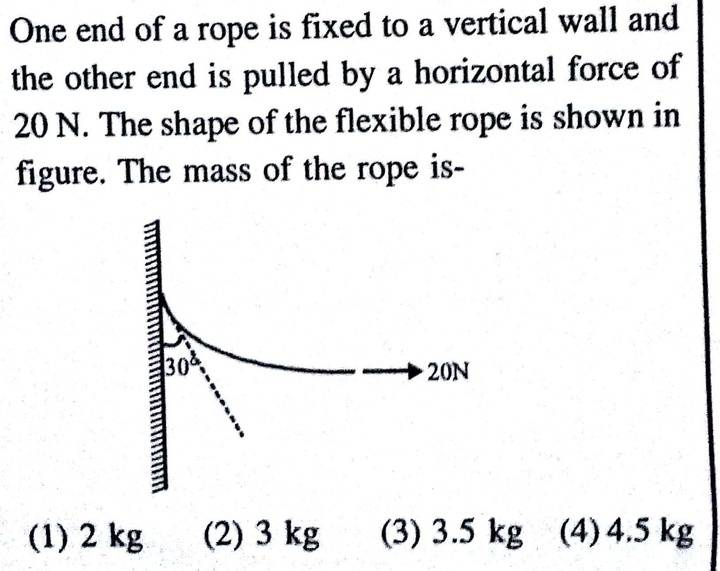# Find mass of a rope pulled by a force

## Homework Statement## The Attempt at a Solution

The force F exerted by the wall on the left end of the rope will make an angle 30° with the vertical . If we do the force balance ,

F sin30° = 20
Fcos30° = Mg

This gives M = 2√3 Kg . This gives 3.5 Kg . But answer given is option 4) . What is the mistake ?

Edit : There is a mistake in the answer key . Correct option is 3) .

Last edited: